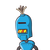# A number with 100 digits is given. The first two digits of that number are 2 and 9 . How many digits has a square of that number

A number with 100 digits is given. The first two digits of that number are 2 and 9 . How many digits has a square of that number?

### 1 thought on “A number with 100 digits is given. The first two digits of that number are 2 and 9 . How many digits has a square of that number”

1.Given : a number   with 100 digits

The first two digits of that number are 2 and 9

To Find : How many digits has a square of that number

29yyyy________

Hence its less than

300000_________

3 with 99  0’s

and greater than

2000000_______

2 with 99  0’s

Square of  3 with 99 0’s will be

9000000_______

9 with 198  0’s

Hence 199  digits

Square of  2 with 99 0’s will be

4000000_______

4 with 198  0’s

Hence 199  digits

Hence square of that number

is greater than a number of 199 digits also lesser than  a number of 199 digits

Hence number must be of 199 digits

so square of that number will have 199 Digits